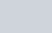•
•
•
•
•
•
•
•

# PROPERTIES AND SOLUTIONS OF TRIANGLE

Here, there are some key concepts regarding the properties and solutions of triangles. these are very important for the students who preparing for IIT-JEE and other equivalent exams.

## POINTS TO BE REMEMBERED

### LAWS OF SINE OR SINE RULE

The sides of a triangle are proportional to the sines of opposite angles i.e, In an ∆ABC,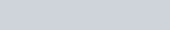Where k is some constant.

### LAWS OF COSINES OR COSINES RULE–

In any ∆ABC , We have### PROJECTION FORMULA –

In any ∆ABC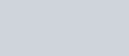### LAWS OF TANGENT OR TANGENT RULES (Napier’s Analogy)### HALF ANGLE FORMULAE OR SEMI-SUM FORMULAE### AREA OF TRIANGLE –

The area of any triangle ABC can be given by: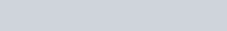### HERON’S FORMULA –

In a ∆ABC , if a+b+c = 2s, then it’s area can given by: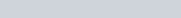### CIRCUMCIRCLE OF A TRIANGLEIn any triangle ABC,

a).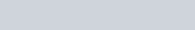b).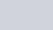Everywhere  means area

### INCIRCLE OF A TRIANGLEIn any triangle ABC,
a).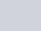b).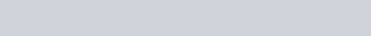c).d).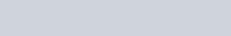### EXCRIBED CIRCLE OF A TRIANGLEIn any triangle ABC
a).b).c).d).### ORTHOCENTRE OF A TRIANGLEThe triangle formed by joining the points F, E, and D is called the pedal triangle. In any triangle ABC,

a). The distance of the orthocentre from the vertices of the triangle ABC is-

Distance from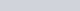Distance from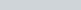Distance fromb). Distance of the orthocentre from the sides of the triangle ABC is-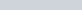,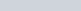,#### POINTS TO BE NOTED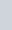2). Area of a pedal triangle is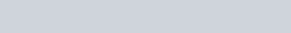3). Length of the median AD, BE, and CF of the ∆ABC. is given by-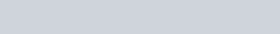BE =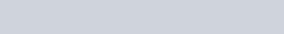CF =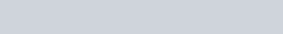4). Length of the angle bisector through vertex A=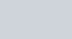Through vertex B =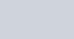Through vertex C =5). Length of the altitude from;

From vertex A =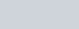From vertex B =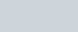From vertex C =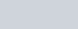6). Distance between circumcentre and the orthocentre =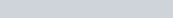7). Distance between circumcentre and incentre is=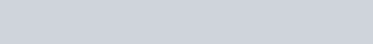8). Distance between incentre and the orthocentre =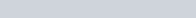9). Distance between circumcentre and centroid =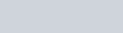10). If circumcentre, centroid, and orthocentre are collinear and G divides OO’ in the ratio of 1:2

11). If I1, I2, and I3 are the center of the escribed circles opposite to the angle A, B, and C respectively.  And O is the orthocentre. Then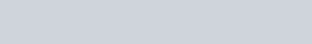#### POINTS TO BE NOTED

1). Area of the cyclic quadrilateral ABCD is =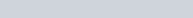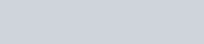3). CosB =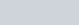and similarly other angles.

### PTOLEMY THEOREM –

#### REGULAR POLYGON

##### POINTS TO BE NOTED

1). If a regular polygon has n sides, then the sum of its internal angle is (n-2)π and each angle is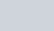2). In a regular polygon the centroid, the circumcentre, and incentre are the same.

3). Area of the regular polygon =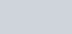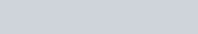Where n is the no of sides of the regular polygon, R is the radius of circumscribing circle, and r is the radius of the incircle of a polygon.

4). Radius of circumscribing circle =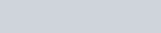Where a is the length of the side of the regular polygon of n sides.

5). Radius of inscribed circles r=